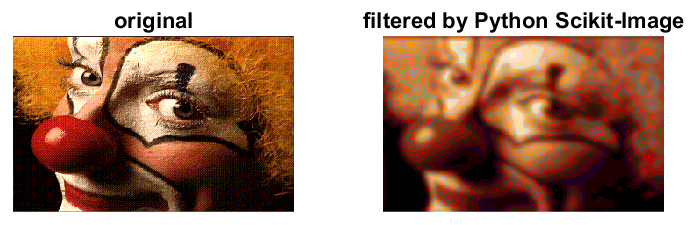Matlab matrices to / from Python

Related:

Passing N-dimensional arrays to/from Python from Matlab is straightforward with Matlab ≥ R2018b. Matlab understands list(), dict(), set(), and other Python classes including Numpy ndarray and Xarray.

Matlab → Python

Using image_proc.m:Filter an image in Python and display in Matlab

Matlab ↔ xarray

These functions are used to access the auroral model GLOW from Matlab via glow.m.

function M = xarray2mat(V)
M = double(py.numpy.asfortranarray(V));
end

function I = xarrayind2vector(V,key)
% convert xarray index to Matlab 1-D matrix

I = cell2mat(cell(V.indexes{key}.values.tolist));

end